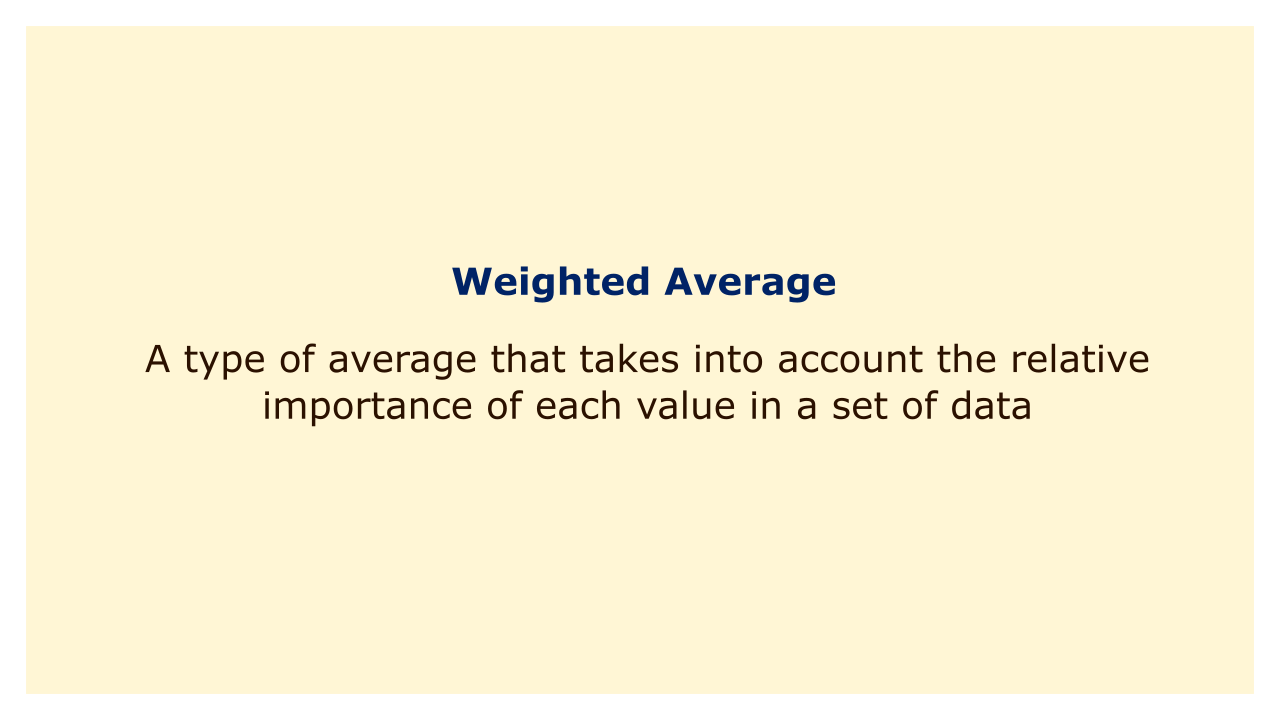# Weighted AverageImage: Moneybestpal.com

### A weighted average is a type of average that takes into account the relative importance of each value in a set of data. The test will carry greater weight in deciding your final mark than the quiz.

For instance, if the test counts for 40% of your grade and the quiz counts for 10% of your grade. In a weighted average, values with higher weights are given more weight, and values with lower weights are given less weight.

To calculate a weighted average, you need to multiply each value by its corresponding weight and then add up the products. The sum of the products is then divided by the sum of the weights to get the weighted average. For example, suppose you have three grades: a test worth 40%, a quiz worth 10%, and a homework worth 50%. Your grades are 80, 90, and 70 respectively.

To find your weighted average, you would do the following:
• Multiply each grade by its weight: 80 x 0.4 = 32; 90 x 0.1 = 9; 70 x 0.5 = 35
• Add up the products: 32 + 9 + 35 = 76
• Divide by the sum of the weights: 76 / (0.4 + 0.1 + 0.5) = 76 / 1 = 76
Therefore, your weighted average is 76.

If you want to compare various sets of data with various scales or units, a weighted average may be helpful. You can use a weighted average to translate two students' scores into a single scale, for instance, if you wish to compare the performance of students who took separate courses with distinct grading systems. In a frequency distribution, where each value is given a frequency or count, the central tendency can also be determined using a weighted average.

A basic or arithmetic average is determined by adding up all the values and dividing by the total number of values. A weighted average is distinct from this. A simple average does not account for each value's proportional frequency or relevance. Your basic average, for instance, would be 80 if you had four grades: two tests worth 50% each, two quizzes at 25% each, and your grades were 80, 90, 60, and 70, respectively:
• Add up all the grades: 80 + 90 + 60 + 70 = 300
• Divide by the number of grades: 300 / 4 = 75
Therefore, your simple average is 75.

This does not, however, indicate that the tests are weighted more heavily in deciding your final grade than the quizzes. Your weighted average would be:
• Multiply each grade by its weight: 80 x 0.5 = 40; 90 x 0.5 = 45; 60 x 0.25 = 15; 70 x 0.25 = 17.5
• Add up the products: 40 + 45 +15 + 17.5 = 117.5
• Divide by the sum of the weights: 117.5 / (0.5 + 0.5 + 0.25 + 0.25) = 117.5 /1.5 = 78.33
Therefore, your weighted average is 78.33.

As you can see, because it gives more weight to your higher test scores, your weighted average is higher than your simple average.

The relative relevance or frequency of each value in a set of data is taken into account by a weighted average, which is a sort of average. You must multiply each value by the corresponding weight before adding the results to determine the weighted average. The weighted average is then calculated by dividing the sum of the products by the sum of the weights.
Tags